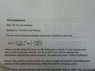# One-Dimensional distribution of Molecular Velocity Questioin

ramsharmjarm
1. Homework Statement [/b]

Calculate the function for N2 at 300k

d(vx)= (m/2pikT)^1/2 exp(-(mv^2/2kT)

I have to be able to use this equation for varying velocity from -1500 m/s to 1500 m/s

## The Attempt at a Solution

Boltzmann constant = 1.3806503 × 10-23 m2 kg s-2 K-1
N2 mass= 0.0280 kg

So I am plugging these 2 values in the equation, but i keep getting 0 when i do the exp(mv^2/2kt part). e to the power of such a high number gives me 0 and that high number comes from my denominator being such as small value, so I am no sure what to do.

I have uploaded question formula picture incase its not clear.

#### Attachments

•IMG_0003.jpg
48.1 KB · Views: 361